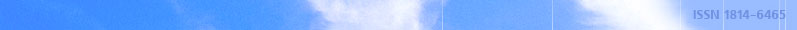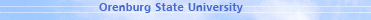november 30, 2021 RU/EN
 Headings of Vestnik Pedagogics Psychology Other

 Search Author Article

# 2014, № 9 (170)

Vaulin S.D., Kartasheva M.A. THE METHODS FOR MODELING OF PERFECT GAS IN AN ANNULAR NOZZLESThe methods for modeling of flows of perfect gas in external expansions annular nozzles without an external contour are researched. The flows scheme in external expansions annular nozzle with short-cut center body is considered. The versions of using of finite-difference methods for calculation flows in subsonic, transonic and supersonic fields of flow are proposed. On basis of proposed calculations methods are developed computational algorithms for modeling of gasdynamic and thrust characteristics of external expansions annular nozzle.Key words: external expansions annular nozzle, computational domain, relaxational method, finite-difference scheme.

References:

1. Kartashev, A.L. Mathematical modeling of flows in annular nozzles / A.L. Kartashev, M.A. Kartasheva. — Chelyabinsk, Yuzhno-Uralskiy Gos. Univ., 2011. — 158 p.

2. Godunov, S.K. Differential method of numerical calculation of discontinuous solutions of equations of fluid dynamic / S.K. Godunov // Mathematical collection, 1959. — № 47, Vol. 3. — P. 271–306.

3. Godunov, S.K. Difference scheme / S.K. Godunov, V.S. Ryabenky. — М.: Nauka, 1973. — 400 p.

4. Kolgan, V.P. Application of a principle of minimum values of a derivative to construction of finite-difference schemes for calculation of discontinuous solutions of gas dynamics / V.P. Kolgan // Uch. Zap. TsAGI [TsAGI Science Journal], 1972. — Vol.3, № 6. — P. 68–77.

5. Kolgan, V.P. Finite-difference scheme for calculation of two dimensional discontinuous solutions of unsteady gas dynamics / V.P. Kolgan // Uch. Zap. TsAGI [TsAGI Science Journal], 1975. — Vol.6, № 1. — P. 9–14.

6. Tillyaeva, N.I. Research of ability of V.P. Kolgan's modification of S.K. Godunov's numerical scheme, keeping approximation for arbitrary computational grid / N.I. Tillyaeva // Engineering report CIAM, no. 9860. — Moscow, 1982. — 46 p.

7. Computational solution of multidimensional problems of gas dynamics / S.K. Godunov, A.V. Zabrodin, M.Ja. Ivanov, etc. — M.: Nauka, 1976. — 400 p.

8. Ivanov, M.Ya. Method of "transparent" calculation of two dimensional and three dimensional supersonic flows / M.Ya. Ivanov, A.N. Kraiko, N.V. Mikhailov // Journal of computer mathematics and mathematical physics, 1972. — Vol.12, № 2. — P. 441–463.

9. Ivanov, M.Ya. Method of "transparent" calculation of two dimensional and three dimensional supersonic flows / M.Ya. Ivanov, A.N. Kraiko // Journal of computer mathematics and mathematical physics, 1972. — Vol.12, № 3. — P. 805–813.

10. Two-phase mono- and polydisperse flows of gas with particles / L.E. Sternin, B.N. Maslov, A.A. Shrajber, etc. — M.: Mashinostroenie, 1980. — 176 p.

11. Godunov, S.K. Difference scheme for two dimensional unsteady problems of gasdynamics and calculation of flow with separated shock wave / S.K. Godunov, A.V. Zabrodin, G.P. Prokopov // Journal of computer mathematics and mathematical physics, 1961. — Vol. 1, № 6. — P. 1020–1050.

12. Ivanov, M.Ya. Numerical solution of direct problem of mixed flow in nozzle / M.Ya. Ivanov, A.N. Kraiko // Izvestiya RAN. Fluid Dynamics, 1969. — № 5. — P. 77–83.

13. Ivanov, M.Ya. Calculation of mixed flow of gas in nozzles / M.Ya. Ivanov, A.N. Kraiko // Proceeding of section of numerical methods of gas dynamics of second International colloquium of explosionўs gas dynamics and reaction systems. — М.: Computer Center of USSR Academy of Sciences, 1971. — P. 3–26.

14. Research off conditions of controllable plate annular nozzle / Yu.V. Gora, L.Z. Grebenyuk, N.D. Kovalenko etc. // Dynamics of pump systems. — Kiev: Naukova dumka, 1980. — P. 137–144.

15. Gora, Yu.V. Numerical research of flow of ideal gas in transonic field of annular nozzle with mobile outer contour / Yu.V. Gora // Dynamics of pump systems. — Kiev: Naukova dumka, 1980. — P. 152.Editor-in-chief Sergey Aleksandrovich MIROSHNIKOV